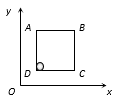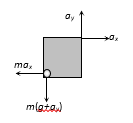Pseudo force
Question

# A solid sphere of mass 2 kg is resting inside a cube as shown in the figure. The cube is moving with a velocity $\stackrel{\to }{\mathrm{V}}=\left(5\text{\hspace{0.17em}}\stackrel{^}{\mathrm{i}}+2\mathrm{t}\text{\hspace{0.17em}}\stackrel{^}{\mathrm{j}}\right)\mathrm{m}/\mathrm{s}$ . Here t is the time in second. All surface are smooth. The sphere is at rest with respect to the cube. What is the total force exerted by the sphere on the cube. (Take g = 10 $\mathrm{m}/{\mathrm{s}}^{2}$)Moderate
Solution

## $\stackrel{\to }{\mathrm{F}}=\mathrm{m}{\mathrm{a}}_{\mathrm{x}}\stackrel{^}{\mathrm{i}}+\mathrm{m}\left(\mathrm{g}+{\mathrm{a}}_{\mathrm{y}}\right)\stackrel{^}{\mathrm{j}}$$\therefore |\stackrel{\to }{\mathrm{F}}|=\mathrm{m}\sqrt{{\mathrm{a}}_{\mathrm{x}}^{2}+{\left(\mathrm{g}+{\mathrm{a}}_{\mathrm{y}}\right)}^{2}}=24\text{ }\mathrm{N}$Get Instant Solutions